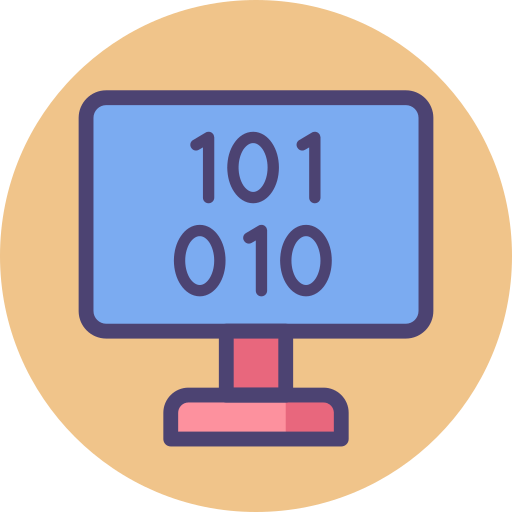# Data Representation II

Welcome to class!

In today’s class, we will be talking more about data representation. Enjoy the class!

### Data Representation IINumber System defines a set of values used to represent ‘quantity’.

• Base or Radix: The total number of digits available in a number system.
• Decimal number system:

There are 10 digits i.e. 1, 2, 3, 4, 5, 6, 7, 8, 9 available in the decimal number system. It is known as the Base 10 system. The value of a digit in a number depends upon its position in the number e.g. the number 546 in this system is represented as (546)10
546 = (4*102) + (8*101) + (6*100)

• Binary number system:

The Binary Number System contains 2 unique digits 0 and 1. It is known as Base 2 system.

• Octal number system:

There are 8 unique digits available in the octal number system. These are 0, 1, 2, 3, 4, 5, 6, 7. Thus, any number formed is the combination of these digits. It is known as the Base 8 system.

There are 16 unique digits available in the Hexadecimal number system. These are 0, 1, 2, 3, 4, 5, 6, 7, 8, A, B, C, D, E, F where A denotes 10, B denotes 11………, F denotes 15. Thus any number formed is a combination of these digits. It is known as the Base 16 system.

#### Converting Decimal to Binary, Octal and Hexadecimal

• ##### Integer part

Remainder method

1. Divide the decimal number by the base of the target number system that is, to convert decimal to binary, divide the decimal number with 2 (the base of binary number system), 8 for octal and 16 for hexadecimal.
2. Note the remainder separately as the first digit from the right. In case of hexadecimal, if the remainder exceeds 9, convert the remainder into equivalent hexadecimal form. E.g., if the remainder is 10 then note the remainder as A.
3. Continually repeat the process of dividing until the quotient is zero and keep writing the remainders after each step of division.
4. Finally, when no more division can occur, write down the remainders in reverse order.
##### Fractional part
1. Multiply the fractional part by the value of the new base.
2. Record the integer part if it exists, else record 0.
3. Repeat step 1 with the result of the previous multiplication and then step 2, until the fractional part becomes 0. In case of infinite calculations, generally, 6 digits are taken.

#### Converting Binary, Octal and Hexadecimal to Decimal

The method used for conversion of a binary, octal and hexadecimal number to decimal number involves each digit of the binary, octal or hexadecimal number to be multiplied by its weighted position, and then each of the weighted value is added to get the decimal number.

Example:

 Binary Number 1 1 0 1 0 Weight of each bit 24 22 22 21 20 Weighted Value 24 * 1 23 * 1 22 * 0 21 * 1 20 * 0 Solved Multiplication 16 8 0 2 0

Sum of weight of all bits = 16 + 8 +0 + 2 + 0 = 26
Thus, the decimal equivalent of (11010)2 is (26)10

#### Converting Between Octal and Hexadecimal

Steps of conversion

1. Convert each octal digit to 3-bit binary form
2. Combine all the 3-bit binary numbers.
3. Segregate the binary numbers into 4-bit binary form by starting the first number from the right bit (LSB) towards the number on the left bit (MSB).
4. Finally, convert these 4-bit blocks into their respective hexadecimal symbols.
###### Evaluation
1. A single unit which is composed of a small group of bits is known as (a) bit (b) bug (c) flag (d) byte
2. BCD stands for (a) Binary Coded Decimal (b) Binary Coded Digitals (c) Binary Characters Decimals (d) Binary Conducting Decimals
3. The system in which fractions are written by extending binary rotation is called (a) fixed-point representation (b) floating-point representation (c) binary digits representation (d) single rotation representation
4. ‘megabytes’ of computer storage capacity consists of (a) one million bytes (b) two million bytes (c) three million bytes (d) four million bytes
5. In BCD code, maximum possible characters set size is (a) character set of 64 (b) character set of 84 (c) character set of 94 (d) character set of 104

In our next class, we will be talking about Security and Ethics.  We hope you enjoyed the class.

Should you have any further question, feel free to ask in the comment section below and trust us to respond as soon as possible.

How Can We Make ClassNotesNG Better - CLICK to Tell Us💃